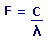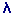Frequency and Wavelength CalculatorF= Frequency C= Speed Of Light= Wavelength

Enter value and click on unit. Result will be displayed.

or or or or or

 Results:

 Web www.calculatoredge.com
 Frequency is a measure of the number of occurrences of a repeating event per unit time.

SI unit of frequency is Hertz (Hz), named after the German physicist Heinrich Hertz. One hertz means one cycle per second, 100 Hz means one hundred cycles per second, and so on

Wavelength is the distance between repeating units of propagating wave of a given frequency. Wavelength can be represented by symbol Lambda. Examples of wave like phemomena are light, water waves and sound waves.

Wavelength can be expressed in any unit of Length such as Meters, Feet, Centimeters etc.

The Speed of Light in a vacuum is an important physical constant denoted by the letter C for constant.

In Metric Units, the Speed of Light in vacuum is exactly 299,792,458 metres per second (1,079,252,848.8 km/h), approximate value of 3 × 10^8 m/s. In Imperial Units, the speed of light is about 670,616,629.2 miles per hour or 983,571,056 feet per second, which is about 186,282.397 miles per second, or roughly one foot per nanosecond.LINKSDISCLAIMERCONTACT US